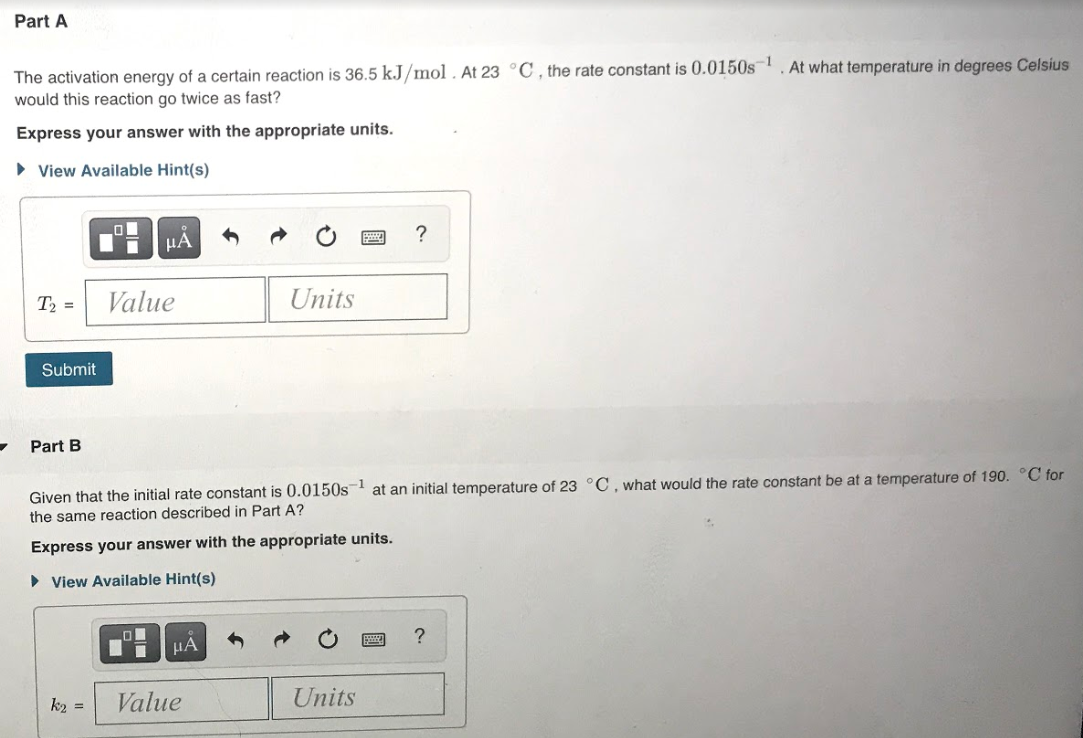# The activation energy of a certain reaction is 36.5 kJ/mol/ At 23 °C, the rate constant is 0.0150s^-1. At what temperature in degrees Celsius would this reaction go twice as fast? Express your answer with the appropriate units. Given that the initial rate constant is 0.0150s^-1 at an initial temperature of 23 °C, what would the rate constant be at a temperature of 190. °C for the same reaction described in Part A? Express your answer with the appropriate units.